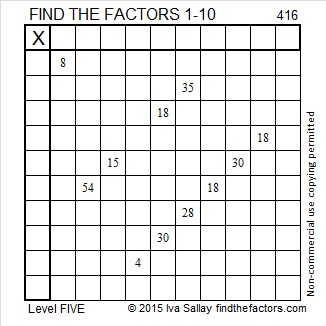# 416 and Level 5

416 is part of many Pythagorean triples. The smallest one is [160-384-416]. The largest one is the only primitive of the lot: [416-43,263-43,265]. Even though I find these triples incredibly easy to find, you can rest assured that you will probably go your entire life without ever having to know either of them!Print the puzzles or type the factors on this excel file: 10 Factors 2015-03-02

• 416 is a composite number.
• Prime factorization: 416 = 2 x 2 x 2 x 2 x 2 x 13, which can be written 416 = (2^5) x 13
• The exponents in the prime factorization are 5 and 1. Adding one to each and multiplying we get (5 + 1)(1 + 1) = 6 x 2 = 12. Therefore 416 has exactly 12 factors.
• Factors of 416: 1, 2, 4, 8, 13, 16, 26, 32, 52, 104, 208, 416
• Factor pairs: 416 = 1 x 416, 2 x 208, 4 x 104, 8 x 52, 13 x 32, or 16 x 26
• Taking the factor pair with the largest square number factor, we get √416 = (√16)(√26) = 4√26 ≈ 20.3961